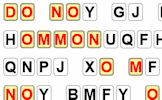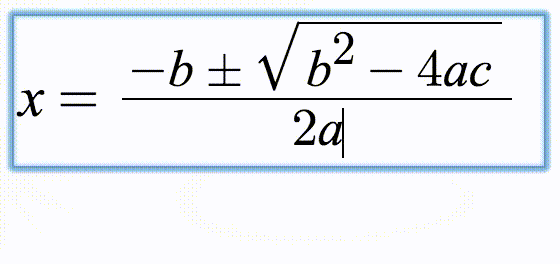# Algebragons

## Find the missing expressions in these partly completed algebraic arithmagon puzzles.

##### MenuArithmagonsLevel 1Level 2Level 3Level 4Level 5HelpMore Algebra

Complete the algebragons. The expressions in the rectangles are the sums of the linear expressions in the adjacent circles.

 $$x$$ $$3x$$ $$2x$$+ $$2x+1$$ $$5x-3$$ $$3x+2$$+ $$2x$$$$x-5$$ $$3x+4$$+ $$9-3x$$ $$x+4$$$$6x+4$$+ $$1-x$$$$x+6$$$$6x+1$$ +$$9-3x$$ $$10$$ $$16-2x$$+
Check

This is Algebragons level 1. You can also try:
Level 2 Level 3 Level 4 Level 5

## Instructions

Try your best to answer the questions above. Type your answers into the boxes provided leaving no spaces. As you work through the exercise regularly click the "check" button. If you have any wrong answers, do your best to do corrections but if there is anything you don't understand, please ask your teacher for help.

When you have got all of the questions correct you may want to print out this page and paste it into your exercise book. If you keep your work in an ePortfolio you could take a screen shot of your answers and paste that into your Maths file.

## Transum.org

This web site contains over a thousand free mathematical activities for teachers and pupils. Click here to go to the main page which links to all of the resources available.## More Activities:

Mathematicians are not the people who find Maths easy; they are the people who enjoy how mystifying, puzzling and hard it is. Are you a mathematician?

Comment recorded on the 24 May 'Starter of the Day' page by Ruth Seward, Hagley Park Sports College:

"Find the starters wonderful; students enjoy them and often want to use the idea generated by the starter in other parts of the lesson. Keep up the good work"

Comment recorded on the 11 January 'Starter of the Day' page by S Johnson, The King John School:

"We recently had an afternoon on accelerated learning.This linked really well and prompted a discussion about learning styles and short term memory."

Each month a newsletter is published containing details of the new additions to the Transum website and a new puzzle of the month.

The newsletter is then duplicated as a podcast which is available on the major delivery networks. You can listen to the podcast while you are commuting, exercising or relaxing.

Transum breaking news is available on Twitter @Transum and if that's not enough there is also a Transum Facebook page.

#### Code CrackerLearn the basic techniques for cracking codes then practise them using this interactive challenge. There are three levels of difficulty and many different messages to decipher.

There are answers to this exercise but they are available in this space to teachers, tutors and parents who have logged in to their Transum subscription on this computer.

A Transum subscription unlocks the answers to the online exercises, quizzes and puzzles. It also provides the teacher with access to quality external links on each of the Transum Topic pages and the facility to add to the collection themselves.

Subscribers can manage class lists, lesson plans and assessment data in the Class Admin application and have access to reports of the Transum Trophies earned by class members.

Subscribe

## Go Maths

Learning and understanding Mathematics, at every level, requires learner engagement. Mathematics is not a spectator sport. Sometimes traditional teaching fails to actively involve students. One way to address the problem is through the use of interactive activities and this web site provides many of those. The Go Maths page is an alphabetical list of free activities designed for students in Secondary/High school.

## Maths Map

Are you looking for something specific? An exercise to supplement the topic you are studying at school at the moment perhaps. Navigate using our Maths Map to find exercises, puzzles and Maths lesson starters grouped by topic.

## Teachers

If you found this activity useful don't forget to record it in your scheme of work or learning management system. The short URL, ready to be copied and pasted, is as follows:

Alternatively, if you use Google Classroom, all you have to do is click on the green icon below in order to add this activity to one of your classes.

It may be worth remembering that if Transum.org should go offline for whatever reason, there are mirror sites at Transum.com and Transum.info that contain most of the resources that are available here on Transum.org.

When planning to use technology in your lesson always have a plan B!

Saturday, June 18, 2022

"

"

Do you have any comments? It is always useful to receive feedback and helps make this free resource even more useful for those learning Mathematics anywhere in the world. Click here to enter your comments.For All:

© Transum Mathematics :: This activity can be found online at:
www.Transum.org/go/?Num=889

## Description of Levels

CloseArithmagons - The same sort of puzzle but with numbers not algebra.

Level 1 - The expressions in the rectangles are the sums of the linear expressions in the adjacent circles.

Level 2 - The expressions in the rectangles are the sums of the linear and quadratic expressions in the adjacent circles.

Level 3 - The expressions in the rectangles are the products of the monomial expressions in the adjacent circles.

Level 4 - The expressions in the rectangles are the products of the binomial expressions in the adjacent circles.

Level 5 - The expressions in the rectangles are the products of the mixed (including fractions) expressions in the adjacent circles.

Fractionagons - Different topic, same structure.

More Algebra including lesson Starters, visual aids, investigations and self-marking exercises.

Answers to this exercise are available lower down this page when you are logged in to your Transum account. If you don’t yet have a Transum subscription one can be very quickly set up if you are a teacher, tutor or parent.

## Mathematical Notation

Use the ^ key to type in a power or index then the right arrow or tab key to end the power.

For example: Type 3x^2 to get 3x2.

Use the forward slash / to type a fraction then the right arrow or tab key to end the fraction.

For example: Type 1/2 to get ½.

Fractions should be given in their lowest terms.

Use brackets if the numerator has more than one term.

Don't wait until you have finished the exercise before you click on the 'Check' button. Click it often as you work through the questions to see if you are answering them correctly. You can double-click the 'Check' button to make it float at the bottom of your screen.

Answers to this exercise are available lower down this page when you are logged in to your Transum account. If you don’t yet have a Transum subscription one can be very quickly set up if you are a teacher, tutor or parent.

Here are some suggestions for how you may solve the puzzles in which only the three expressions in the rectangles are given (the last question in levels three and above):

### For novice learners

1. Guess an expression for the top circle
2. From that calculate the values that would be in the bottom two circles
3. Do these two values sum to the bottom rectangle? If so finish.
4. If not is their sum too high or too low? Adjust the top circle value accordingly and go back to (2)

Replace 'sum' with 'product' if the operation is multiplication.

1. Let y stand for the top circle expression
2. From that find expressions for the bottom two circles
3. Make an equation from the bottom two circles and the bottom rectangle
4. Make y the subject of that equation. Now you have the expression for the top circle.

### A great 'trick'

This was found by Ann Roberts, a Transum subscriber, who wrote with this method of solving an arithmagon with 18, 15 and 11 in the rectangles. It also works for algebraic expressions.

1. Add the numbers in the rectangles: 18 + 15 + 11 = 44. This is the same as adding the numbers in the blank circles twice.
2. Divide this sum by two: 44 ÷ 2 = 22. This is now the same as adding the numbers in the blank circles once.
3. Finally: 15 is the sum of the bottom white circles so 15 + top white circle = 22

### Typing Mathematical Notation

These exercises use MathQuill, a web formula editor designed to make typing Maths easy and beautiful. Watch the animation below to see how common mathematical notation can be created using your keyboard.Close